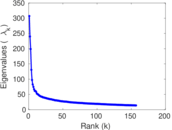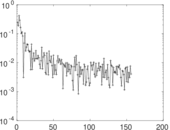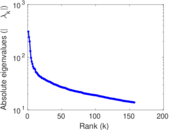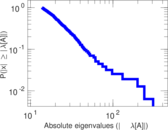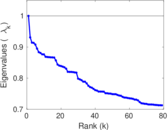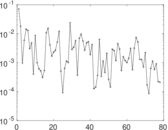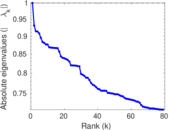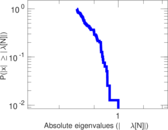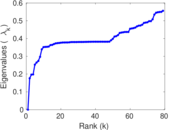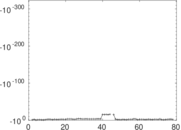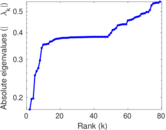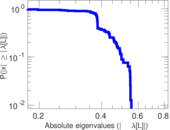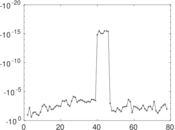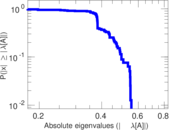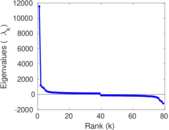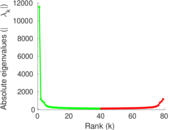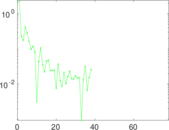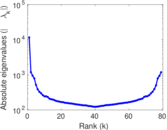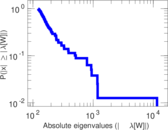# MovieLens user–item

This bipartite network consists of 72,000 users who applied tags to 10,000 movies from http://movielens.umn.edu/. Left nodes represent users and right nodes represent movies. An edge connects a user with a movie he has assigned a tag to.

 Code `Mui` Internal name `movielens-10m_ui` Name MovieLens user–item Data source http://www.grouplens.org/node/73 AvailabilityDataset is available for download Consistency checkDataset passed all tests Category Interaction network Node meaning User, movie Edge meaning Tag assignment Network formatBipartite, undirected Edge typeUnweighted, multiple edges Temporal dataEdges are annotated with timestamps

## Statistics

 Size n = 11,610 Left size n1 = 4,009 Right size n2 = 7,601 Volume m = 95,580 Unique edge count m̿ = 55,484 Wedge count s = 10,527,036 Claw count z = 3,111,884,288 Cross count x = 940,679,536,237 Square count q = 7,490,391 4-Tour count T4 = 102,152,360 Maximum degree dmax = 6,012 Maximum left degree d1max = 6,012 Maximum right degree d2max = 308 Average degree d = 16.465 1 Average left degree d1 = 23.841 4 Average right degree d2 = 12.574 7 Fill p = 0.001 820 79 Average edge multiplicity m̃ = 1.722 66 Size of LCC N = 11,557 Diameter δ = 9 50-Percentile effective diameter δ0.5 = 3.304 04 90-Percentile effective diameter δ0.9 = 4.618 16 Median distance δM = 4 Mean distance δm = 3.768 25 Gini coefficient G = 0.746 738 Balanced inequality ratio P = 0.209 128 Left balanced inequality ratio P1 = 0.125 811 Right balanced inequality ratio P2 = 0.250 983 Relative edge distribution entropy Her = 0.849 226 Power law exponent γ = 1.817 63 Tail power law exponent γt = 2.401 00 Tail power law exponent with p γ3 = 2.401 00 p-value p = 0.929 000 Left tail power law exponent with p γ3,1 = 1.651 00 Left p-value p1 = 0.000 00 Right tail power law exponent with p γ3,2 = 3.321 00 Right p-value p2 = 0.002 000 00 Degree assortativity ρ = −0.221 845 Degree assortativity p-value pρ = 0.000 00 Spectral norm α = 307.003 Algebraic connectivity a = 0.176 189 Spectral separation |λ1[A] / λ2[A]| = 1.279 70 Controllability C = 5,981 Relative controllability Cr = 0.515 159

## Plots

### Fruchterman–Reingold graph drawing### Degree distribution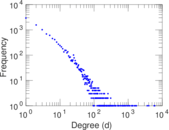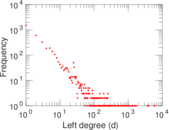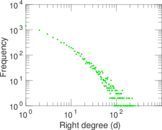### Cumulative degree distribution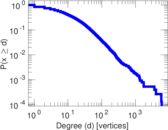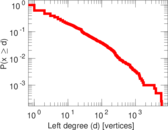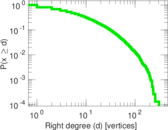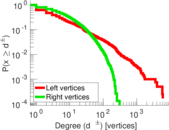### Lorenz curve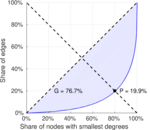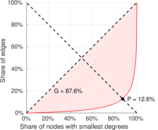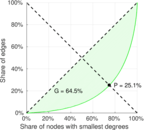### Spectral distribution of the adjacency matrix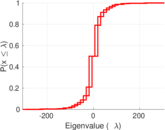### Spectral distribution of the normalized adjacency matrix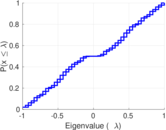### Spectral distribution of the Laplacian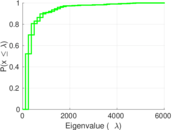### Spectral graph drawing based on the adjacency matrix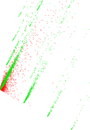### Spectral graph drawing based on the Laplacian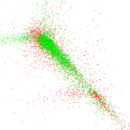### Spectral graph drawing based on the normalized adjacency matrix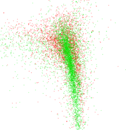### Degree assortativity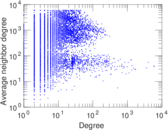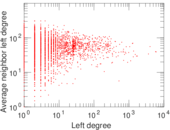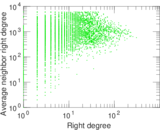### Zipf plot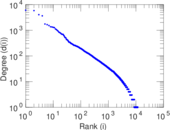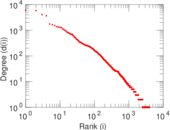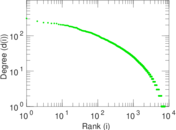### Hop distribution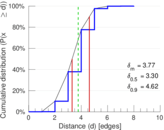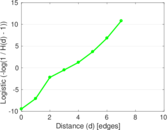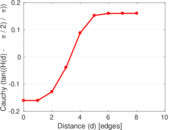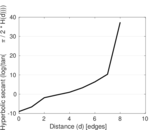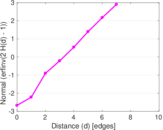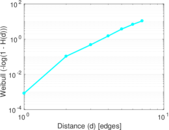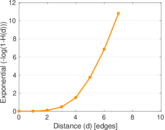### Double Laplacian graph drawing### Delaunay graph drawing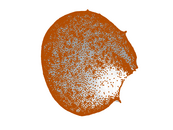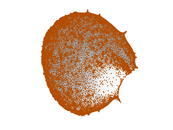### Edge weight/multiplicity distribution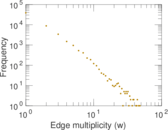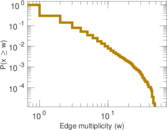### Temporal distribution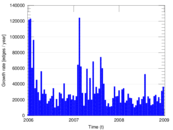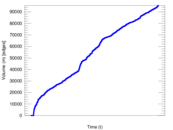### Temporal hop distribution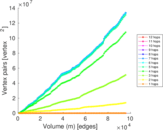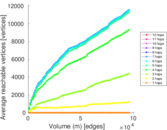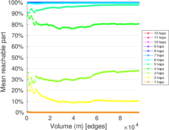### Diameter/density evolution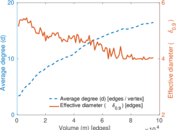### Inter-event distribution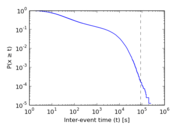### Node-level inter-event distribution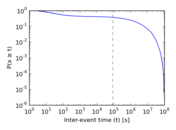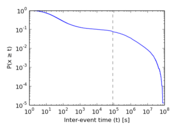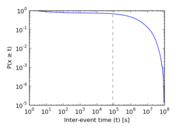### Matrix decompositions plots